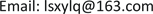﻿ Wick型随机mKdV方程带多参量的广义的有理指数函数解 A Generalized Rational-Exponent Function Solution with Multi-Parameter for Wick-Type Stochastic mKdV Equation

Vol.06 No.09(2017), Article ID:22973,7 pages
10.12677/AAM.2017.69128

A Generalized Rational-Exponent Function Solution with Multi-Parameter for Wick-Type Stochastic mKdV Equation

Qing Liu

Institute of Optics and Electronics, School of Engineering, Lishui University, Lishui ZhejiangReceived: Nov. 22nd, 2017; accepted: Dec. 4th, 2017; published: Dec. 11th, 2017ABSTRACT

With the help of Hermite transformation, white noise theory and a rational-exponent function solution of Riccati equation, a rational-exponent function solution with multiple arbitrary parameters for Wick-type stochastic mKdV equation is obtained. From this rational-exponent function solution, the influence of variety of different parameters on the dynamics behavior of Wick-type stochastic mKdV equation by restricted Riccati equation is showed. It provides a more effective method, which can be used to investigate the influence of external conditions and internal factors on behavior of nonlinear stochastic dynamics model.

Keywords:Wick-Type Stochastic mKdV Equation, Rational-Exponent Function Solution, White Noise, Hermite Transformation

Wick型随机mKdV方程带多参量的广义的有理指数函数解1. 引言

Wadati最先研究了随机偏微分KdV方程和在高斯白噪声条件下KdV方程的孤子色散  ，接着研究了高斯白噪声条件下有阻尼和无阻尼KdV方程  ，随后提出了一种描述波在任意介质传播的非线性偏分方程  。此外，de Bouard   、Debussche   、Konotop  、Printems  、Holden  等人对随机偏微分方程的研究也做大量的工作。

${U}_{t}-{K}_{1}\left(t\right)\diamond \left({U}_{xxx}-6{U}^{\diamond 2}\diamond {U}_{x}\right)-4{K}_{2}\left(t\right)\diamond {U}_{x}+H\left(t\right)\diamond \left(U+x{U}_{x}\right)=0,$ (1)

2. 主要步骤

${A}^{\diamond }\left(t,x,{\partial }_{t},{\nabla }_{x},U,\omega \right)=0,$ (2)

$\stackrel{˜}{A}\left(t,x,{\partial }_{t},{\nabla }_{x},\stackrel{˜}{U},{z}_{1},{z}_{2},\cdots \right)=0,$ (3)

$\stackrel{˜}{U}\left(t,x,z\right)=u\left(t,x,z\right)=u\left(\xi \right),\text{\hspace{0.17em}}\xi =f\left(t,z\right)x+g\left(t,z\right),$ (4)

$A\left(u,{u}_{\xi },{u}_{\xi \xi },{u}_{\xi \xi \xi },\cdots \right)=0,$ (5)

$u\left(\xi \right)=\underset{i=0}{\overset{n}{\Sigma }}{a}_{i}\left(t,z\right){F}^{i}\left(\xi \right),$ (6)

$k{F}_{\xi }=q+p{F}^{2}\left(\xi \right),$ (7)

$u\left(\xi \right)={a}_{0}\left(t,z\right)+{a}_{1}\left(t,z\right)F\left(\xi \right)+\cdots +{a}_{n}\left(t,z\right){F}^{n}\left(\xi \right).$ (8)

3. Wick型随机mKdV方程的有理指数函数解

$\begin{array}{l}{\stackrel{˜}{U}}_{t}\left(t,x,z\right)-{\stackrel{˜}{K}}_{1}\left(t,z\right)\left({\stackrel{˜}{U}}_{xxx}\left(t,x,z\right)-6{\stackrel{˜}{U}}^{2}\left(t,x,z\right){\stackrel{˜}{U}}_{x}\left(t,x,z\right)\right)\\ -4{\stackrel{˜}{K}}_{2}\left(t,z\right){\stackrel{˜}{U}}_{x}\left(t,x,z\right)+\stackrel{˜}{H}\left(t,z\right)\left(\stackrel{˜}{U}\left(t,x,z\right)+x{\stackrel{˜}{U}}_{x}\left(t,x,z\right)\right)=0,\end{array}$ (9)

$u\left(t,x,z\right)=\stackrel{˜}{U}\left(t,x,z\right),\text{\hspace{0.17em}}H\left(t,z\right)=\stackrel{˜}{H}\left(t,z\right),\text{\hspace{0.17em}}K\left(t,z\right)={\stackrel{˜}{K}}_{i}\left(t,z\right)\text{\hspace{0.17em}}\left(i=1,2\right),$

$u=u\left(\xi \right),\text{\hspace{0.17em}}\xi =f\left(t,z\right)x+g\left(t,z\right),$ (10)

$u\left(\xi \right)={a}_{0}\left(t,z\right)+{a}_{1}\left(t,z\right)F\left(\xi \right)+\cdots +{a}_{n}\left(t,z\right){F}^{n}\left(\xi \right)$ (11)

$k{F}_{\xi }=q+p{F}^{2}\left(\xi \right),$ (12)

$F\left(\xi \right)=\frac{{A}_{1}\left({A}_{1}{\text{e}}^{\xi }+{A}_{-1}\right)}{{B}_{1}\left({A}_{1}{\text{e}}^{\xi }-{A}_{-1}\right)},$ (13)

$\left({f}_{t}x+{g}_{t}\right){u}_{\xi }-{K}_{1}\left({f}^{3}{u}_{\xi \xi \xi }-6f{u}^{2}{u}_{\xi }\right)-4{K}_{2}f{u}_{\xi }+H\left(u+fx{u}_{\xi }\right)=0.$ (14)

$u\left(\xi \right)={a}_{0}\left(t,z\right)+{a}_{1}\left(t,z\right)F\left(\xi \right).$ (15)

${F}_{\xi \xi }=-\frac{1}{2}F-\frac{p}{2q}{F}^{3},$ (16)

${F}_{\xi \xi \xi }=-\frac{1}{4\sqrt{-pq}}\left(q+4p{F}^{2}+\frac{3}{q}{p}^{2}{F}^{4}\right).$ (17)

$\begin{array}{l}3{K}_{1}\sqrt{-\frac{p}{q}}f{a}_{1}\left(\frac{p}{4q}{f}^{2}-{a}_{1}^{2}\right){F}^{4}+6f{K}_{1}\sqrt{-\frac{p}{q}}{a}_{0}{a}_{1}^{2}{F}^{3}\end{array}$ $+\sqrt{-\frac{p}{q}}{a}_{1}\left(-3{K}_{1}f{a}_{1}^{2}\frac{q}{p}+{K}_{1}{f}^{3}+\frac{1}{2}{g}_{t}+3{K}_{1}f{a}_{0}^{2}-2{K}_{2}f\right){F}^{2}$ $+\frac{1}{2}\sqrt{-\frac{p}{q}}{a}_{1}\left({f}_{t}+Hf\right)x{F}^{2}+\left({a}_{1t}+H{a}_{1}+6k{K}_{1}\sqrt{-\frac{q}{p}}f{a}_{0}{a}_{1}^{2}\right)F$ $+\frac{1}{2}\sqrt{-\frac{q}{p}}{a}_{1}\left({f}_{t}+Hf\right)x+\left({a}_{0t}+H{a}_{0}-2{K}_{2}\sqrt{-\frac{q}{p}}f{a}_{1}+\frac{1}{2}\sqrt{-\frac{q}{p}}{a}_{1}{g}_{t}+3{K}_{1}\sqrt{-\frac{q}{p}}f{a}_{0}^{2}{a}_{1}$ $+\frac{1}{4}{K}_{1}\sqrt{-\frac{q}{p}}{f}^{3}{a}_{1}\right)=0.$ (18)

${F}^{i},x{F}^{i}\text{\hspace{0.17em}}\left(i=0,1,2,3,4\right)$ 所有系数分别都为零，得到下列一组关于未知量 ${a}_{0},{a}_{1}$ 的代数方程，即

$3{K}_{1}\sqrt{-\frac{p}{q}}f{a}_{1}\left(\frac{p}{4q}{f}^{2}-{a}_{1}^{2}\right)=0,$ (19)

$6f{K}_{1}\sqrt{-\frac{p}{q}}{a}_{0}{a}_{1}^{2}=0,$ (20)

$\sqrt{-\frac{p}{q}}{a}_{1}\left(-3{K}_{1}f{a}_{1}^{2}\frac{q}{p}+{K}_{1}{f}^{3}+\frac{1}{2}{g}_{t}+3{K}_{1}f{a}_{0}^{2}-2{K}_{2}f\right)=0,$ (21)

$\frac{1}{2}\sqrt{-\frac{p}{q}}{a}_{1}\left({f}_{t}+Hf\right)=0,$ (22)

$\left({a}_{1t}+H{a}_{1}+6k{K}_{1}\sqrt{-\frac{q}{p}}f{a}_{0}{a}_{1}^{2}\right)=0,$ (23)

$\frac{1}{2}\sqrt{-\frac{q}{p}}{a}_{1}\left({f}_{t}+Hf\right)=0,$ (24)

$\left({a}_{0t}+H{a}_{0}-2{K}_{2}\sqrt{-\frac{q}{p}}f{a}_{1}+\frac{1}{2}\sqrt{-\frac{q}{p}}{a}_{1}{g}_{t}+3{K}_{1}\sqrt{-\frac{q}{p}}f{a}_{0}^{2}{a}_{1}+\frac{1}{4}{K}_{1}\sqrt{-\frac{q}{p}}{f}^{3}{a}_{1}\right)=0.$ (25)

${a}_{0}\left(t,z\right)=0.$ (26)

$3{K}_{1}\sqrt{-\frac{p}{q}}f{a}_{1}\left(\frac{p}{4q}{f}^{2}-{a}_{1}^{2}\right)=0,$ (27)

$\frac{1}{2}\sqrt{-\frac{p}{q}}{a}_{1}\left({f}_{t}+Hf\right)=0,$ (28)

$\frac{1}{2}\sqrt{-\frac{q}{p}}{a}_{1}\left({f}_{t}+Hf\right)=0,$ (29)

${a}_{1t}+H{a}_{1}=0,$ (30)

$\sqrt{-\frac{q}{p}}{a}_{1}\left(-2{K}_{2}f+\frac{1}{2}{g}_{t}+\frac{1}{4}{K}_{1}{f}^{3}\right)=0,$ (31)

$\sqrt{-\frac{p}{q}}{a}_{1}\left(-3{K}_{1}f{a}_{1}^{2}\frac{q}{p}+{K}_{1}{f}^{3}+\frac{1}{2}{g}_{t}-2{K}_{2}f\right)=0.$ (32)

$f\left(t,z\right)=C{}_{1}\text{e}{}^{\left(-{\int }_{}^{t}H\left(s,z\right)\text{d}s\right)},$ (33)

${a}_{1}\left(t,z\right)=±\sqrt{\frac{p}{4q}}{C}_{1}{\text{e}}^{\left(-{\int }_{}^{t}H\left(s,z\right)\text{d}s\right)},$ (34)

$g\left(t,z\right)={\int }_{}^{t}\left(-4{C}_{2}{K}_{2}\left(s,z\right){\text{e}}^{\left(-{\int }_{}^{s}H\left(\tau ,z\right)\text{d}\tau \right)}+\frac{1}{2}{C}_{2}^{3}{K}_{1}\left(s,z\right){\text{e}}^{\left(-3{\int }_{}^{s}H\left(\tau ,z\right)\text{d}\tau \right)}\right)\text{d}s+{C}_{3},$ (35)

$u\left(t,x,z\right)=±\sqrt{\frac{p}{4q}}{C}_{1}{\text{e}}^{\left(-{\int }_{}^{t}H\left(s,z\right)\text{d}s\right)}F\left(\xi \right),$ (36)

$\xi =C{}_{1}x{\text{e}}^{\left(-{\int }_{}^{t}H\left(s,z\right)\text{d}s\right)}+{\int }_{}^{t}\left(-4{C}_{2}{K}_{2}\left(s,z\right){\text{e}}^{\left(-{\int }_{}^{s}H\left(\tau ,z\right)\text{d}\tau \right)}$ $+\frac{1}{2}{C}_{2}^{3}{K}_{1}\left(s,z\right){\text{e}}^{\left(-3{\int }_{}^{s}H\left(\tau ,z\right)\text{d}\tau \right)}\right)\text{\hspace{0.17em}}\text{d}s+{C}_{3}$ (37)

${K}_{1}\left(t\right)={b}_{1}W\left(t\right),\text{\hspace{0.17em}}{K}_{2}\left(t\right)={b}_{2}W\left(t\right),\text{\hspace{0.17em}}H\left(t\right)=h\left(t\right)+{b}_{3}W\left(t\right),$ (38)

${K}_{1}\left(t,z\right)={b}_{1}\stackrel{˜}{W}\left(t,z\right),\text{\hspace{0.17em}}{K}_{2}\left(t,z\right)={b}_{2}\stackrel{˜}{W}\left(t,z\right),\text{\hspace{0.17em}}H\left(t,z\right)=h\left(t\right)+{b}_{3}\stackrel{˜}{W}\left(t,z\right).$

$U\left(t,x\right)=±\sqrt{\frac{p}{4q}}{C}_{1}{\text{e}}^{\diamond \left(-{\int }_{}^{t}H\left(s\right)\text{d}s\right)}{F}^{\diamond }\left(\overline{\xi }\right),$ (39)

$\overline{\xi }={C}_{1}x{\text{e}}^{\diamond \left(-{\int }_{}^{t}H\left(s\right)\text{d}s\right)}+{\int }_{}^{t}\left(-4{C}_{2}{K}_{2}\left(s\right)\diamond {\text{e}}^{\diamond \left(-{\int }_{}^{s}H\left(\tau \right)\text{d}\tau \right)}$ $+\frac{1}{2}{C}_{2}^{3}{K}_{1}\left(s\right)\diamond {\text{e}}^{\diamond \left(-3{\int }_{}^{s}H\left(\tau \right)\text{d}\tau \right)}\right)\text{\hspace{0.17em}}\text{d}s+{C}_{3}.$ (40)

${\text{e}}^{\diamond \left(B\left(t\right)\right)}={\text{e}}^{\left(B\left(t\right)-\frac{1}{2}{t}^{2}\right)},\text{ }{\int }_{R}\psi \left(t\right)\diamond W\left(t\right)\text{d}t={\int }_{R}\psi \left(t\right)\delta B\left(t\right),\text{ }W\left(t\right)=\stackrel{˙}{B}\left(t\right),$ (41)

$U\left(t,x\right)=±\sqrt{\frac{p}{4q}}{C}_{1}{\text{e}}^{\left(-{\int }_{}^{t}h\left(s\right)\text{d}s-{b}_{3}B\left(t\right)+\frac{1}{2}{b}_{3}{t}^{2}\right)}F\left(\theta \right)$ (42)

$\theta =x{C}_{1}{\text{e}}^{\left(-{\int }_{}^{t}h\left(s\right)\text{d}s-{b}_{3}B\left(t\right)+\frac{1}{2}{b}_{3}{t}^{2}\right)}+{\int }_{}^{t}\left(-4{C}_{2}{b}_{2}{\text{e}}^{\left(-{\int }_{}^{s}h\left(\tau \right)\text{d}\tau -{b}_{3}B\left(s\right)+\frac{1}{2}{b}_{3}{s}^{2}\right)}$ $+\frac{1}{2}{C}_{2}^{3}{b}_{1}{\text{e}}^{\diamond \left(-3-{\int }_{}^{s}h\left(\tau \right)\text{d}\tau -3{b}_{3}B\left(s\right)+\frac{3}{2}{b}_{3}{s}^{2}\right)}\right)\text{\hspace{0.17em}}\delta B\left(s\right)+{C}_{3}.$ (43)

$\xi =\theta$ ，然后把方程(13)代入方程(42)，在高斯白噪声条件下随机方程(1)一种带有多个任意参量的有理指数函数解被得到

$U\left(t,x\right)=±\sqrt{\frac{p}{4q}}{C}_{1}{\text{e}}^{\left(-{\int }_{}^{t}h\left(s\right)\text{d}s-{b}_{3}B\left(t\right)+\frac{1}{2}{b}_{3}{t}^{2}\right)}\frac{{A}_{1}\left({A}_{1}{\text{e}}^{\theta }+{A}_{-1}\right)}{{B}_{1}\left({A}_{1}{\text{e}}^{\theta }-{A}_{-1}\right)},$ (44)

4. 结论

A Generalized Rational-Exponent Function Solution with Multi-Parameter for Wick-Type Stochastic mKdV Equation[J]. 应用数学进展, 2017, 06(09): 1056-1062. http://dx.doi.org/10.12677/AAM.2017.69128

1. 1. Wadati, M. (1983) Stochastic Korteweg-de Vries Equation. Journal of the Physical Society of Japan, 52, 2642-2648.
https://doi.org/10.1143/JPSJ.52.2642

2. 2. Wadati, M. and Akutsu, Y. (1984) Stochastic Korteweg-de Vries Equation with and without Damping. Journal of the Physical Society of Japan, 53, 3342-3350.
https://doi.org/10.1143/JPSJ.53.3342

3. 3. Wadati, M. (1990) Deformation of Solitons in Random Media. Journal of the Physical Society of Japan, 59, 4201-4203.
https://doi.org/10.1143/JPSJ.59.4201

4. 4. Bouard, A.D. and Debussche, A. (1998) On the Stochastic Korteweg-de Vries Equation. Journal of Functional Analysis, 154, 215-251.
https://doi.org/10.1006/jfan.1997.3184

5. 5. Bouard, A.D. and Debussche, A. (1999) White Noise Driven Korteweg-de Vries Equation. Journal of Functional Analysis, 169, 532-258.
https://doi.org/10.1006/jfan.1999.3484

6. 6. Debussche, A. and Printems, J. (1999) Numerical Simulation of the Stochastic Korteweg-de Vries Equation. Physica D, 134, 200-226.
https://doi.org/10.1016/S0167-2789(99)00072-X

7. 7. Debussche, A. and Printems, J. (2001) Effect of a Localized Random Forcing Term on the Korteweg-de Vries Equation. Journal of Computational Analysis and Applications, 3, 183-206.
https://doi.org/10.1023/A:1011596026830

8. 8. Konotop, V.V. and Vźquez, L. (1994) Nonlinear Random Waves. World Scientific, Singapore.
https://doi.org/10.1142/2320

9. 9. Printems, J. (1999) The Stochastic Korteweg-de Vries Equation in L(R2). Journal of Differential Equations, 153, 338-373.
https://doi.org/10.1006/jdeq.1998.3548

10. 10. Holden, H., Øsendal, B., Ubøe, J. and Zhang, T. (1996) Stochastic Partial Differential Equations. BirhKäuser, Boston.
https://doi.org/10.1007/978-1-4684-9215-6

11. 11. Xie, Y.C. (2003) Exact Solutions for Stochastic KdV Equations. Physics Letters A, 310, 161-167.
https://doi.org/10.1016/S0375-9601(03)00265-2

12. 12. Xie, Y.C. (2004) Exact Solutions of the Wick-Type Sto-chastic Kadomtsev-Petviashvili Equations. Chaos, Solitons & Fractals, 21, 473-480.
https://doi.org/10.1016/j.chaos.2003.12.058

13. 13. Wei, C.M., Ren, Y.H. and Xia, Z.Q. (2005) Symmetry Reduc-tions and Soliton-Like Solutions for Stochastic MKdV Equation. Chaos, Solitons & Fractals, 26, 1507-1513.
https://doi.org/10.1016/j.chaos.2005.04.018

14. 14. Dai, C.Q. and Chen, J.L. (2009) Exact Solutions of (2+1)-Dimensional Stochastic Broer-Kaup Equation. Physics Letters A, 373, 1218-1225.
https://doi.org/10.1016/j.physleta.2009.02.018

15. 15. Dai, C.Q. and Zhang, J.F. (2009) Application of He’s Exp-function Method to the Stochastic mKdV Equation. International Journal of Nonlinear Sciences and Numerical Simulation, 10, 675-680.
https://doi.org/10.1515/IJNSNS.2009.10.5.675

16. 16. Liu, Q. (2006) Various Types of Exact Solutions for Sto-chastic mKdV Equation via a Modified Mapping Method. Europhysics Letters, 74, 377-383.
https://doi.org/10.1209/epl/i2005-10556-5

17. 17. Liu, Q. (2007) Some Exact Solutions for Stochastic mKdV equa-tion. Chaos, Solitons & Fractals, 32, 1224-1230.
https://doi.org/10.1016/j.chaos.2005.11.044

18. 18. Liu, Q., Jia, D.L. and Wang, Z.H. (2010) Three Types of Exact Solutions to Wick-Type Generalized Stochastic Korteweg-de Vries Equation. Applied Mathematics and Computation, 215, 3495-3500.
https://doi.org/10.1016/j.amc.2009.10.014

19. 19. Liu, Q., Lan, J.C., Wu, H.Y. and Wang, Z.H. (2010) A Series of Exact Solutions for (2+1)-Dimensional Wick-Type Stochastic Generalized Broer-Kaup System via a Modified Varia-ble-Coefficient Projective Riccati Equation Mapping Method. Applied Mathematics and Computation, 217, 629-638.
https://doi.org/10.1016/j.amc.2010.05.100

20. 20. Liu, Q., Shen, S.Y. and Wang, Z.H. (2013) The Rational Solutions to a Generalized Riccati Equation and Their Application. International Journal of Modern Physics B, 27, 1350013.
https://doi.org/10.1142/S0217979213500136